# ML Aggarwal Solutions for Class 6 Maths Chapter 4 Playing with Numbers

ML Aggarwal Solutions for Class 6 Maths Chapter 4 Playing with Numbers are provided here. By studying the questions of ML Aggarwal Solutions, students can enhance their execution abilities and operate on their strengths and weaknesses. It makes the students feel confident while answering the question paper. All the answers mentioned in the ML Aggarwal Solutions for Class 6 Maths Chapter 4 are valid and modernised, according to the newest syllabus.

Chapter 4 – Playing with Numbers contains the topics related to, Factors and multiples, Number of factors of a given number is finite, Number of multiples of a given number is infinite, Perfect number, Prime factorisation of a number, Highest Common Factor (HCF) of two or more numbers, Least Common Multiple (LCM) of two or more numbers.

## Download the PDF of ML Aggarwal Solutions for Class 6 Maths Chapter 4 Playing with Numbers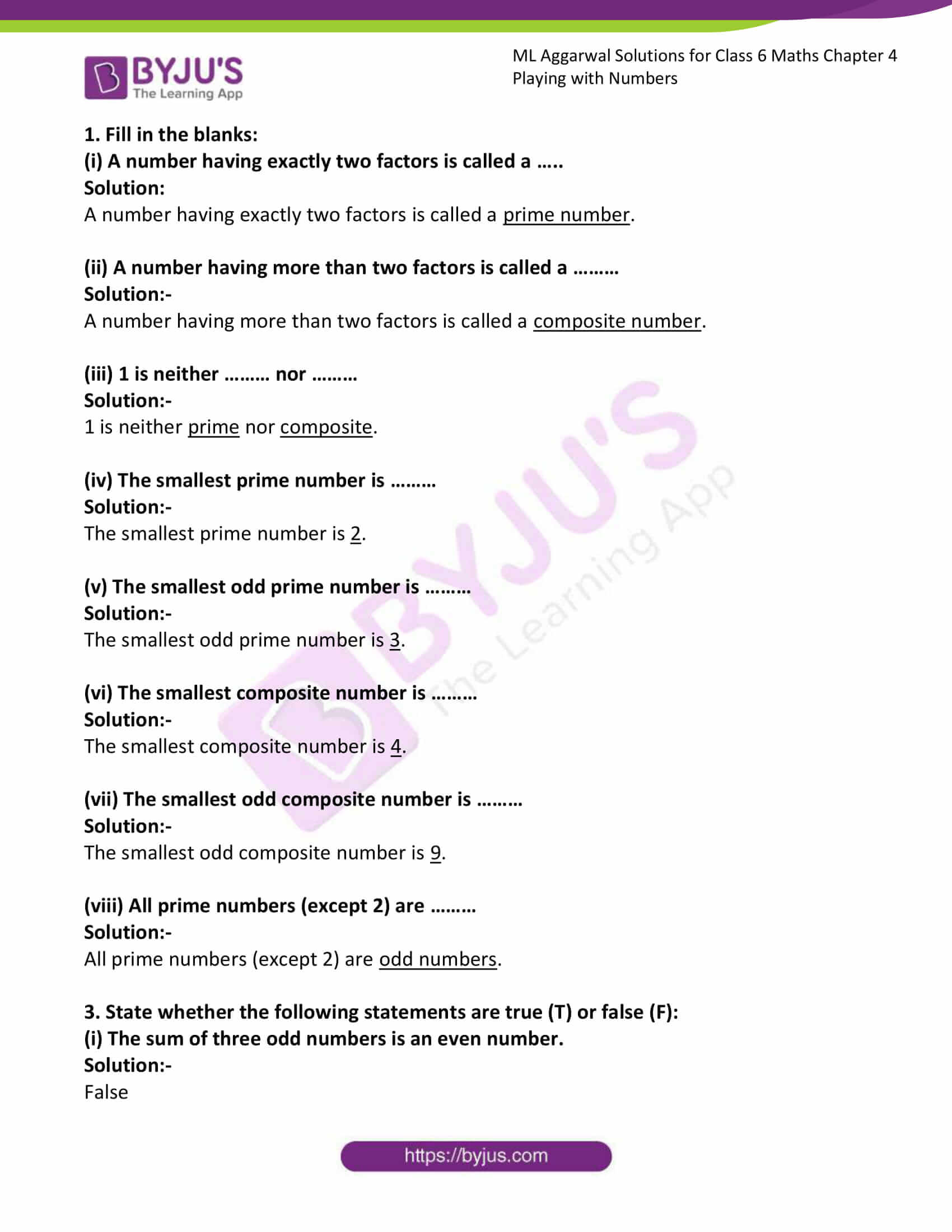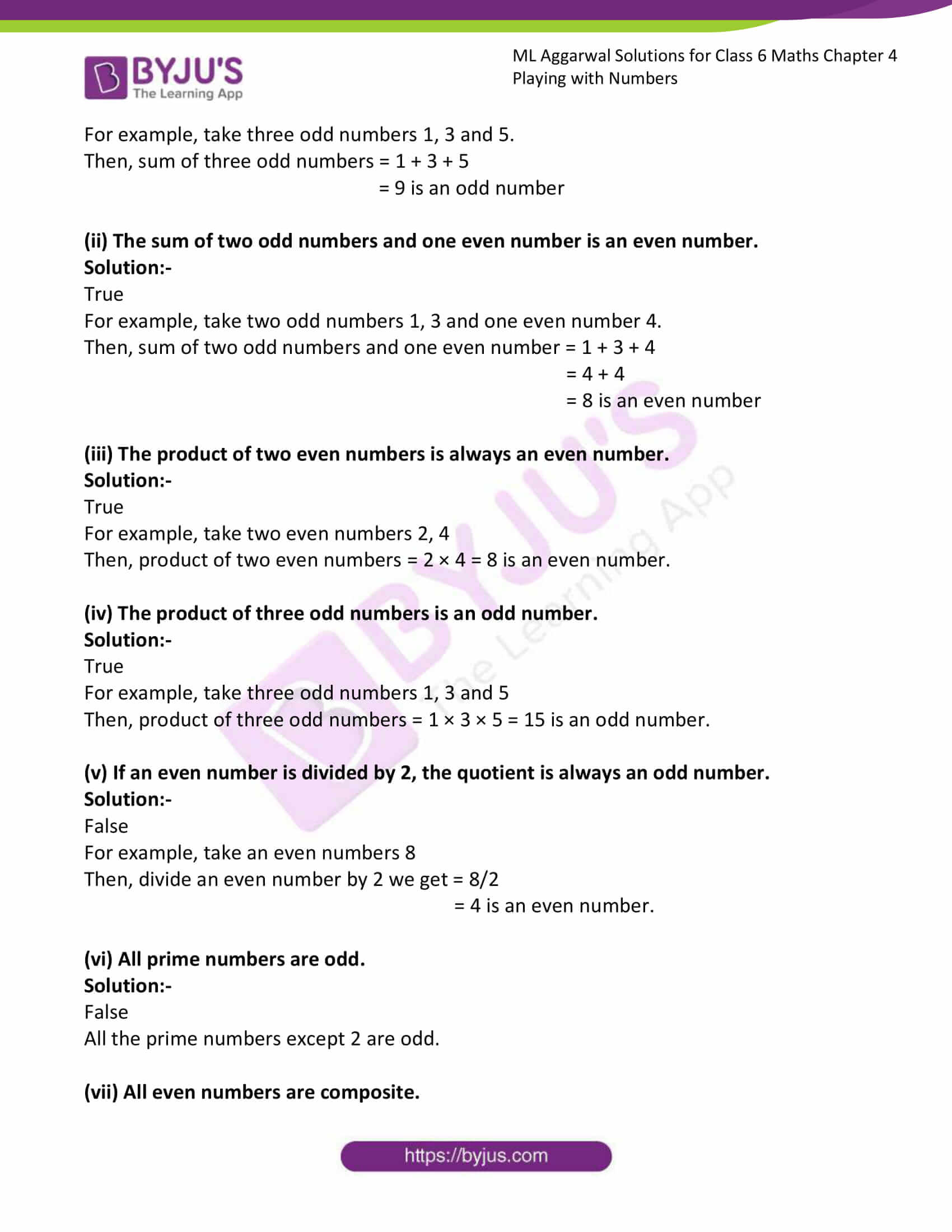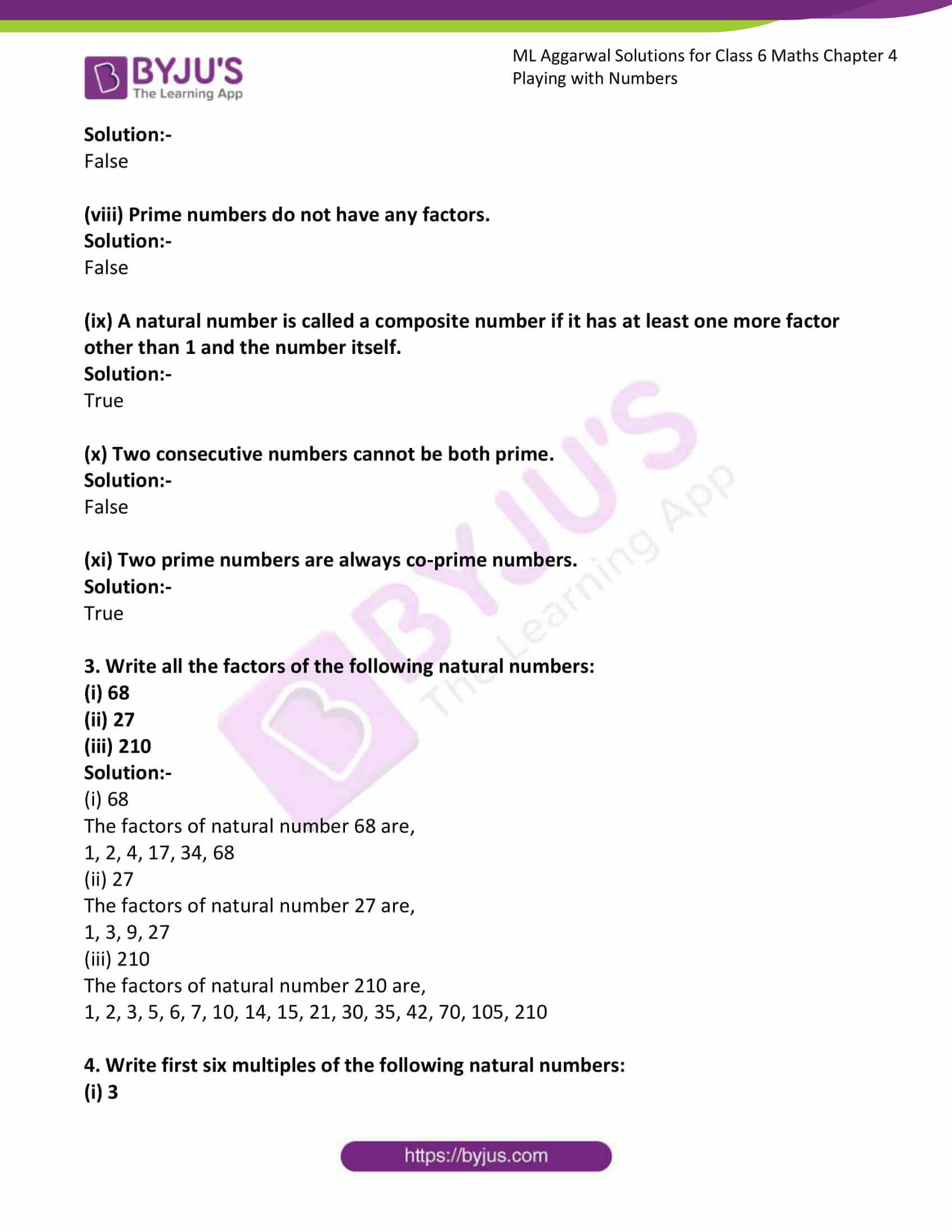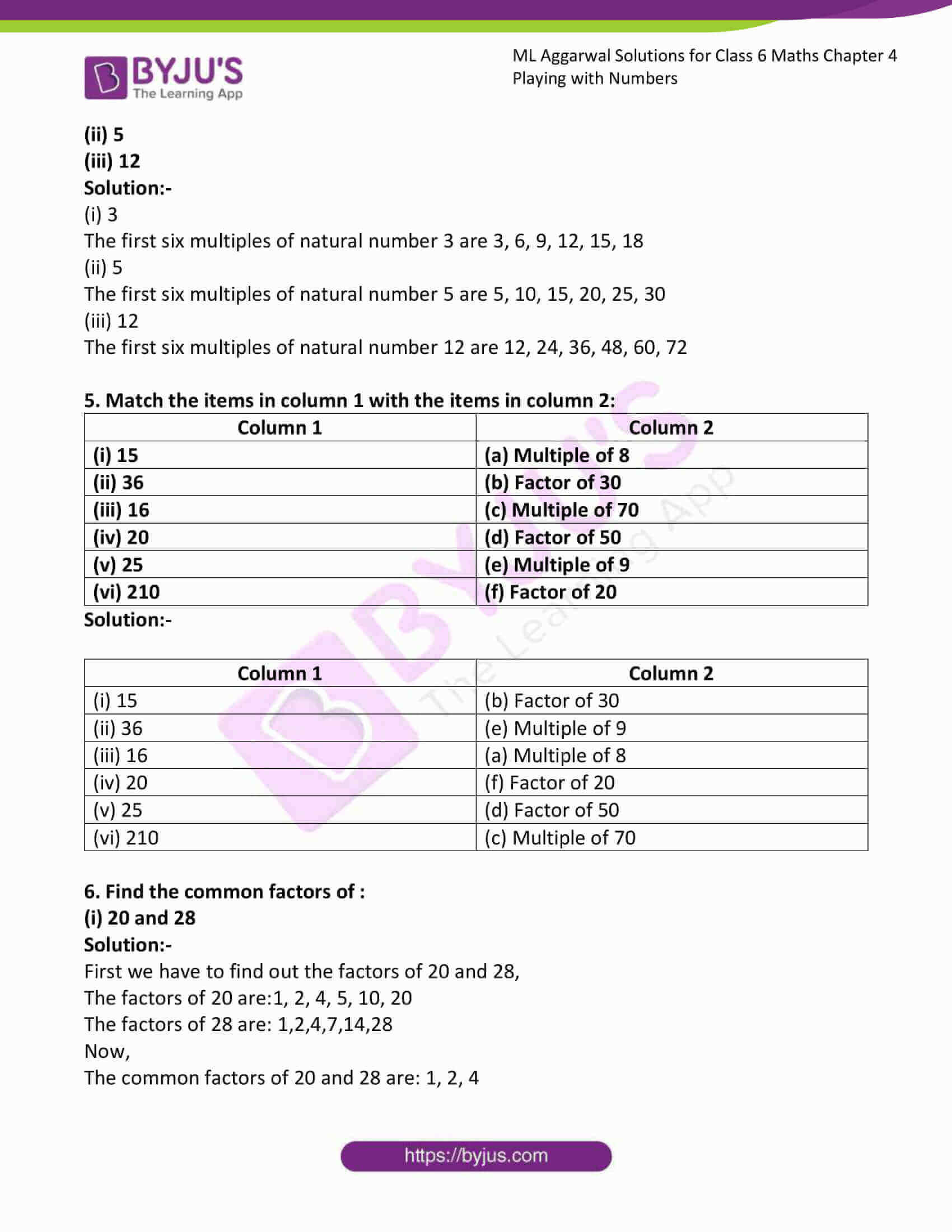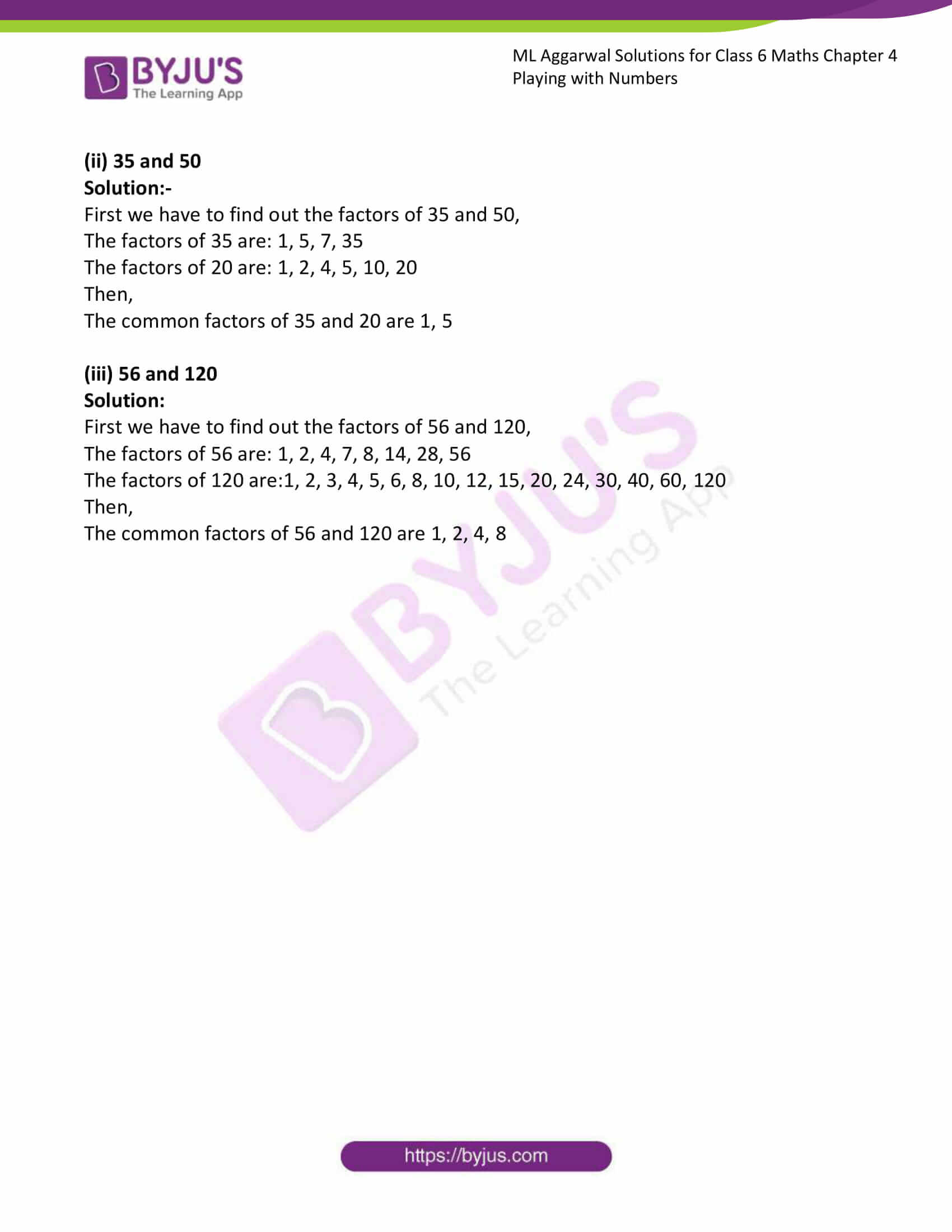### Access answers to ML Aggarwal Solutions for Class 6 Maths Chapter 4 Playing with Numbers

1. Fill in the blanks:
(i) A number having exactly two factors is called a …..

Solution:

A number having exactly two factors is called a prime number.

(ii) A number having more than two factors is called a ………

Solution:-

A number having more than two factors is called a composite number.

(iii) 1 is neither ……… nor ………

Solution:-

1 is neither prime nor composite.

(iv) The smallest prime number is ………

Solution:-

The smallest prime number is 2.

(v) The smallest odd prime number is ………

Solution:-

The smallest odd prime number is 3.

(vi) The smallest composite number is ………

Solution:-

The smallest composite number is 4.

(vii) The smallest odd composite number is ………

Solution:-

The smallest odd composite number is 9.

(viii) All prime numbers (except 2) are ………

Solution:-

All prime numbers (except 2) are odd numbers.

3. State whether the following statements are true (T) or false (F):
(i) The sum of three odd numbers is an even number.

Solution:-

False

For example, take three odd numbers 1, 3 and 5.

Then, sum of three odd numbers = 1 + 3 + 5

= 9 is an odd number

(ii) The sum of two odd numbers and one even number is an even number.

Solution:-

True

For example, take two odd numbers 1, 3 and one even number 4.

Then, sum of two odd numbers and one even number = 1 + 3 + 4

= 4 + 4

= 8 is an even number

(iii) The product of two even numbers is always an even number.

Solution:-

True

For example, take two even numbers 2, 4

Then, product of two even numbers = 2 × 4 = 8 is an even number.

(iv) The product of three odd numbers is an odd number.

Solution:-

True

For example, take three odd numbers 1, 3 and 5

Then, product of three odd numbers = 1 × 3 × 5 = 15 is an odd number.

(v) If an even number is divided by 2, the quotient is always an odd number.

Solution:-

False

For example, take an even numbers 8

Then, divide an even number by 2 we get = 8/2

= 4 is an even number.

(vi) All prime numbers are odd.

Solution:-

False

All the prime numbers except 2 are odd.

(vii) All even numbers are composite.

Solution:-

False

(viii) Prime numbers do not have any factors.

Solution:-

False

(ix) A natural number is called a composite number if it has at least one more factor other than 1 and the number itself.

Solution:-

True

(x) Two consecutive numbers cannot be both prime.

Solution:-

False

(xi) Two prime numbers are always co-prime numbers.

Solution:-

True

3. Write all the factors of the following natural numbers:
(i) 68
(ii) 27
(iii) 210
Solution:-

(i) 68

The factors of natural number 68 are,

1, 2, 4, 17, 34, 68

(ii) 27

The factors of natural number 27 are,

1, 3, 9, 27

(iii) 210

The factors of natural number 210 are,

1, 2, 3, 5, 6, 7, 10, 14, 15, 21, 30, 35, 42, 70, 105, 210

4. Write first six multiples of the following natural numbers:
(i) 3
(ii) 5
(iii) 12
Solution:-

(i) 3

The first six multiples of natural number 3 are 3, 6, 9, 12, 15, 18

(ii) 5

The first six multiples of natural number 5 are 5, 10, 15, 20, 25, 30

(iii) 12

The first six multiples of natural number 12 are 12, 24, 36, 48, 60, 72

5. Match the items in column 1 with the items in column 2:

 Column 1 Column 2 (i) 15 (a) Multiple of 8 (ii) 36 (b) Factor of 30 (iii) 16 (c) Multiple of 70 (iv) 20 (d) Factor of 50 (v) 25 (e) Multiple of 9 (vi) 210 (f) Factor of 20

Solution:-

 Column 1 Column 2 (i) 15 (b) Factor of 30 (ii) 36 (e) Multiple of 9 (iii) 16 (a) Multiple of 8 (iv) 20 (f) Factor of 20 (v) 25 (d) Factor of 50 (vi) 210 (c) Multiple of 70

6. Find the common factors of :
(i) 20 and 28

Solution:-

First we have to find out the factors of 20 and 28,

The factors of 20 are:1, 2, 4, 5, 10, 20

The factors of 28 are: 1,2,4,7,14,28

Now,

The common factors of 20 and 28 are: 1, 2, 4

(ii) 35 and 50
Solution:-

First we have to find out the factors of 35 and 50,

The factors of 35 are: 1, 5, 7, 35

The factors of 20 are: 1, 2, 4, 5, 10, 20

Then,

The common factors of 35 and 20 are 1, 5

(iii) 56 and 120
Solution:

First we have to find out the factors of 56 and 120,

The factors of 56 are: 1, 2, 4, 7, 8, 14, 28, 56

The factors of 120 are:1, 2, 3, 4, 5, 6, 8, 10, 12, 15, 20, 24, 30, 40, 60, 120

Then,

The common factors of 56 and 120 are 1, 2, 4, 8